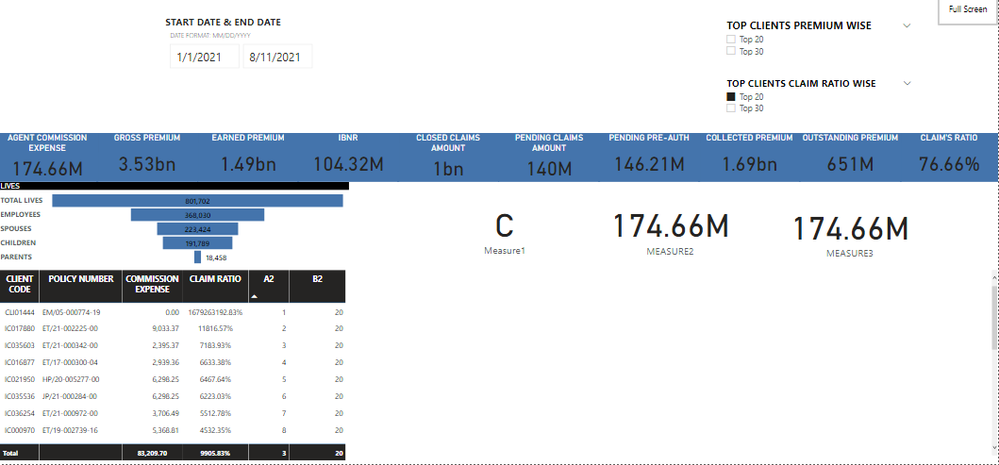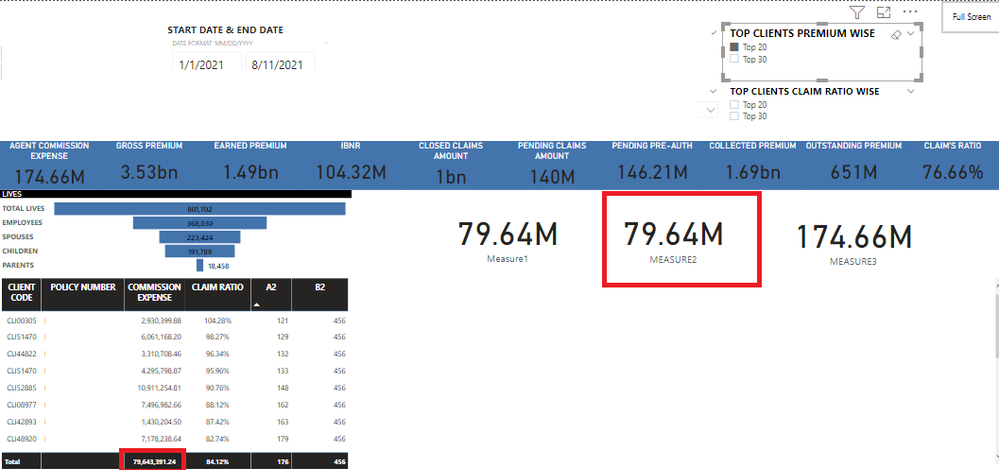cancel
Showing results for
Did you mean:Helper I

## SUM BASED ON RANKX

Hello,

i am unable to get sum using measures based on rankx based on my two filters Top client premium wise and top client claim ratio wise.

below is a screenshot of my dashboardi am trying to get sum of commssion expense with the following dax

``````MEASURE3 =
IF (
[A2]<=SELECTEDVALUE(CLAIMRATIORANK_SLAB[CLAIMRATIORANK]),CALCULATE(SUM(AGENT_COMMISSION[AGENT_COMMISSION]),
FILTER (ALL(DETAILS),[A2]<=SELECTEDVALUE(CLAIMRATIORANK_SLAB[CLAIMRATIORANK]))),
AGENT_COMMISSION[AGENTCOMMBETWEENDATES]
)``````

where A2 is the ranking based on the based on the claim ratio column (which is a measure as well).

i have tried to do the same thing for my top client premium wise filters and it works great by some how it doesnt work on this claim ratio filter

``````MEASURE2 =
IF (
[A1]<=SELECTEDVALUE(GROSSPREMRANK_SLAB[GROSSPREMRANK]),CALCULATE(SUM(AGENT_COMMISSION[AGENT_COMMISSION]),
FILTER (ALL('DETAILS'),[A1]<=SELECTEDVALUE(GROSSPREMRANK_SLAB[GROSSPREMRANK]))),
AGENT_COMMISSION[AGENTCOMMBETWEENDATES]
)``````

this one works fine as its supposed to shown belowany tips on what can i do to fix  DAX measure 3 and get it to work on  top 20 clients claim ratio wise filter

Thanks and regards

shaharyar

1 ACCEPTED SOLUTIONSuper User

@SSWADOOD , If you can get number value from same tbale you are using TOP 20 , 30, Then try to use TOP like

CALCULATE( CALCULATE(SUM(AGENT_COMMISSION[AGENT_COMMISSION])),TOPN( selectedvalue(TOPN[TOPN]),allselected(Date[Date]),CALCULATE(SUM(AGENT_COMMISSION[AGENT_COMMISSION])),DESC), values(GROSSPREMRANK_SLAB[GROSSPREMRANK]))

refer for how it works

2 REPLIES 2Super User

@SSWADOOD , If you can get number value from same tbale you are using TOP 20 , 30, Then try to use TOP like

CALCULATE( CALCULATE(SUM(AGENT_COMMISSION[AGENT_COMMISSION])),TOPN( selectedvalue(TOPN[TOPN]),allselected(Date[Date]),CALCULATE(SUM(AGENT_COMMISSION[AGENT_COMMISSION])),DESC), values(GROSSPREMRANK_SLAB[GROSSPREMRANK]))

refer for how it worksHelper I

thanks @amitchandak for the reply, the video really helped alot. got it to work

``````calculate([agentcommbetweendates],
topN(SELECTEDVALUE(CLAIMRATIORANK_SLAB[CLAIMRATIORANK]),allselected(details[policy_num]),[CLAIM_RATIO],desc)
,values(details[policy_num]))``````

thanks